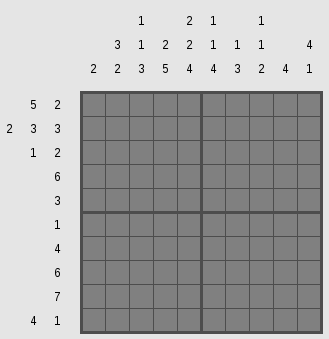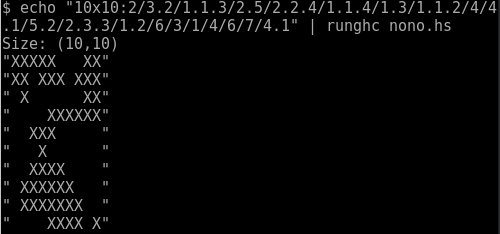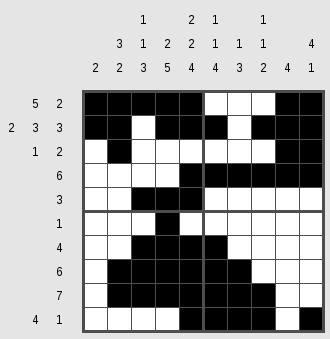# Other articles

1. Published: Wed 18 July 2018

# Prolog Programs, Order of Evaluation, and Functional Equivalents

While teaching Prolog this semester I had a few thoughts about Prolog programs and their equivalent programs in a functional programming language like Haskell.

## The Peano Naturals

The Peano numbers are a unary number system for positive natural numbers where the zero is represented by a Z (or 0, or any atom - it doesn't matter) and every successor of a natural number N is the function S applied to N. We would therefore represent the natural number 3 with the term S(S(S(Z))). To look at it differently: in the Peano axioms, the naturals are defined as the set that contains zero and a closure over the successor function. In haskell we would define the type of the naturals like this:

data N = Z | S N


In Prolog we don't need a type definition for the naturals, as terms are generated without types. Now we want a predicate that checks whether our input is a natural number as we've defined it. The idea is that the term is a natural, iff either the term is a Z or it has an S as its root and its parameter is a natural. In Prolog we would write it intuitively like this:

naturals(z).
naturals(s(X)) :- naturals(X).


And indeed this checks whether our input is a natural number. The query naturals(s(s(z))). returns true while naturals(foo). does not. Writing down this program in Haskell is not very useful since the type system does not allow us to have anything else but a natural as the function parameter but let's pretend:

naturals v = case v of
S x -> naturals x
Z -> True
_ -> False


And of course, calling naturals $S$ S Z in haskell has the correct type and returns true. But this haskell program only corresponds to the case where we actually call the Prolog predicate with a bound parameter. We could call the prolog predicate with a free binding pattern like naturals(X). and get bindings for X for every solution, so every natural number. The corresponding Haskell programm would be:

naturals_f  = [ Z ] ++ map S naturals_f


This might need some explaining: The list corresponds to some notion of non-determinism. In Prolog the non-determinism is baked into the language due to backtracking from failed predicate evaluations and us asking for more answers. The Prolog program essentially says: Z is a solution. Everything with an S is a solution, if the "content" of the S is a solution as well. That's the same for our haskell programm. In our list of solutions we know that Z is in it and for every solution we have, adding an S leads to another one. And evaluating naturals_f starts to calculate the infinite list of natural numbers.

Now consider the following Prolog program where we switched the order of the clauses:

naturals(s(X)) :- naturals(X).
naturals(z).


This still works nicely for the bound case where we call naturals(s(s(z)). But if we now call naturals(X) we quickly get ERROR: Out of local stack. The natural intuition might be that the order of clauses does not matter but it does in this case. Writing down the equivalent Haskell programm we are able to see why:

naturals_f  = map S naturals_f ++ [ Z ]


We have the recursion before we have any solution. Well, the solution is easy: just write the simple case in the first line and the recursive case in the second. But let's consider another problem.

## Repeated Printing

Consider the problem of repeatedly printing a character:

repeat(0, _).
repeat(N, C) :- write(C), NN is N - 1, repeat(NN, C).


This looks very correct and is very wrong.

?- repeat(5, f).
fffff
true ;
ffffffffffffffffffffffffffffffffffffffffffffffffffffffffffffffffffffffffffffffffffffffffffffffffffffffffffffffffffffffffffffffff...


Now we see why it's nice to represent the naturals als Peano numbers. After hitting 0 there's a choice point left and asking for another solution leads to the negatives and printing our character in an infinite loop. So let's augment the second rule to prevent us from going into the negatives.

repeat(0, _).
repeat(N, C) :- N > 0, write(C), NN is N - 1, repeat(NN, C).
% query:
?- repeat(5, f).
fffff
true ;
false.


This looks better but we still have a choice point left over which might be not so nice. The problem is that the matched patterns are not disjoint and after the end of the recursion there's still a pattern left over that fits. We can't encode that N should be larger than 0 in the head of the rule. Remember that we can match naturals larger than 0 (or any other number) in the rule head using Peano numbers - that's why they are so cool.

But how do we fix our problem with the left-over choice point? There are two ways we can do it. We can either introduce a cut or reorder the rules.

% Variant 1a: The order doesn't matter but we introduce a cut
repeat(0, _) :- !.
repeat(N, C) :- N > 0, write(C), NN is N - 1, repeat(NN, C).

% Variant 1b: If we promise to be good and only call repeat with a positive numeric argument, we can even leave out the range check
repeat(0, _) :- !.
repeat(N, C) :- write(C), NN is N - 1, repeat(NN, C).

% Variant 2: The order does matter
repeat(N, C) :- N > 0, write(C), NN is N - 1, repeat(NN, C).
repeat(0, _).


I personally think that the variant without the cut is nicer. So for terminating rules, it might be better to have the "smaller" rule last as to not leave choice-points hanging around. Of course, this only works if the matched terms in the rules are proper subsets of each other so that we actually can order the rules in that fashion. In general, that might not be possible.

## Conclusion

• The order of rules can (in theory) not affect termination. But if our rules are already non-terminating for our query, the order and timeliness of results does matter. Then order does matter.
• Disjoint rule heads are important to not have left-over choice points.
• If rules are terminating but the rule heads are not disjoint, they still should be ordered carefully.
• Implicit conditions (N > 0) can lead to non-obvious non-termination if they are not made explicit.
• By encoding data properties in the term structure we can make some implicit conditions explicit and make rule-heads disjoint.
• Having numerical conditions in rule-heads would be really nice. Maybe some Prolog implementation in the future allows for that.

2. Published: Sun 31 January 2016

# Solving Tatham's Puzzles - Pattern

I've started writing solvers for games of Simon Tatham's Portable Puzzle Collection. Those Problems can usually be reduced to some NP-complete problem. This is quite a fun exercise.

We will be starting with the puzzle "Pattern", also known as Nonograms that we reduce to SAT.Unsolved Nonogram

First to explain the puzzle: The cells of the puzzle can be filled with black or white squares. The numbers on the sides of the rows and colums denote the number of consecutive black squares. Every run of black squares has to be seperated from another run by white squares.

First we want to define a type for our problem.

type PSize = (Int, Int)
type Run = [[Int]]
type Runs = (Run, Run)
data Problem = Problem PSize Runs deriving Show


The puzzle on the side is defined in the Tatham format by the string 10x10:2/3.2/1.1.3/2.5/2.2.4/1.1.4/1.3/1.1.2/4/4.1/5.2/2.3.3/1.2/6/3/1/4/6/7/4.1. It has a size information and then the list of runs. Another format we want to support is the Haskell Runs format which would be ([,[3,2],[1,1,3],[2,5],[2,2,4],[1,1,4],[1,3],[1,1,2],,[4,1]],[[5,2],[2,3,3],[1,2],,,,,,,[4,1]]). This format seperates the runs for rows and columns and ommits the size information.

For the second format we can use Haskell's own read function. For Tatham's format we have to define a parser. I've added type information for most functions which is often needed for code involving read.

import Text.ParserCombinators.ReadP
number' = many1 (choice (map char ['0'..'9']))

r_size = do l <- number'
char 'x'
r <- number'

r_run = do { li <- sepBy number' (char '.'); return $map read li } r_runs :: ReadP [[Int]] r_runs = sepBy r_run (char '/') r_problem :: ReadP Problem r_problem = do size <- r_size; char ':' runs <- r_runs skipSpaces; eof return$ Problem size (take (fst size) runs, drop (fst size) runs)


What do we now do with such a problem? First we want to check it for consistency. If we got size information it should fit the runs and the numbers in the runs (and the spaces between them) shouldn't add up to more than the size.

import qualified Prelude as P (all, (&&), not)
consistent :: Problem -> Bool
consistent (Problem size (xin, yin)) = consistent' size xin P.&& consistent' (swap size) yin
consistent' (w, h) xs = (w == length xs) P.&& P.all (\x -> (sum x) + (length x - 1) <= h) xsCalling our solver

But now on to solving the problem. We will reduce this problem to SAT using the Ersatz library. The encoding of a problem, I guess, is obvious. We say that every cell within the Nonogram is a Variable Bit and a bit being True has the semantics of that field being colored black while False would mean white.

We want to build constraints of single rows or columns and then solve the conjunction of all those row/column-constraints for satisfiability. Therefore we define a function of the type [Int] -> [Bit] -> Bit that takes a list of Integers (the numbers of one column or row) and the list of remaining Bits that do not yet are constrained.

First we begin with the end of the recursion. If no number is left or the remaining number is zero (for empty columns or rows), we want all remaining variables to be False. (we take for to be defined as flip map)

build_runs :: [Int] -> [Bit] -> Bit
build_runs nums vars = case nums of
[] -> all not vars
 -> all not vars
x:xs -> let max_d = length vars - (length xs + sum xs) - x
run   = [not] ++ replicate x id ++ [not]
v     = [false] ++ vars ++ [false]
in any id $for [0..max_d]$ \ d ->
(all not $take d vars) && (all id$ zipWith id run $take (x + 2)$ drop d v)
&& (build_runs xs $drop (x + d + 1) vars)Solved Nonogram The recursion is something to think about a bit. We're given a number of consecutive black squares x and all the variables in the column/row vars. The x Trues can be put anywhere from 0 to max_d where max_d is all the rest of the variables take the minimum necessary space of the remaining number of numbers xs, while all variables before that number of black squares and one after need to be white. For every possible position the rest of the variables need to fulfill the same condition with the rest of the numbers. Now we have to combine all the constraints from a given problem. nono :: (MonadState s m, HasSAT s) => Problem -> m (Map (Int,Int) Bit) nono (Problem (sizex, sizey) (xin, yin)) = do let indices = [(x, y) | x <- [1..sizex], y <- [1..sizey] ] vars' <- replicateM (length indices) exists vars <- return$ Map.fromList $zip indices vars' let getx x = for [1..sizey]$ \y -> (vars ! (x,y))
gety y = for [1..sizex] $\x -> (vars ! (x,y)) xs = zipWith build_runs xin (map getx [1..]) ys = zipWith build_runs yin (map gety [1..]) assert (all id xs && all id ys) return vars  Internally we let Ersatz identify variables by their position in the grid Map (Int,Int) Bit. Now we can solve our problem easily using minisat with Ersatz's native minisat binding. Given a solution, we just format it correctly and give it to the user. main = do input <- getContents let parsed = parse_tat input problem = case parsed of Just p -> p Nothing -> let (xin, yin) = read input :: ([[Int]],[[Int]]) in Problem (length xin, length yin) (xin, yin) putStrLn$ "Size: " ++ (show $problem_size problem) unless (consistent problem)$ do {putStrLn "Problem inconsitent"; exitFailure}

(Satisfied, Just solution) <- solveWith minisat (nono problem)
let sizey = snd $problem_size problem lines = for [1..sizey]$ \i -> filter (\ ((x, y), b) -> y == i) $toList solution conv = map$ \((x,y), b)-> if b then 'X' else ' '
join = foldr (:) ""
forM_ (map (join . conv) lines) print


And we're done.

Find the code here: github.com/maweki/tatham-slv

3. Published: Thu 12 November 2015

# talk at Leipzig's Haskell conference HaL-10

As I said before: I will hold a small talk about dogelang at Leipzig's next Haskell conference HaL-10.

Here's a translation of the abstract (original in German):

dg - The better Haskell is named Python

Who needs typeclasses? Or static type system even? Why have side-effects to be so complicated? And what are monads?

There must be something better!

dg is Python with Haskell syntax. In a short talk (15 minutes) I want to introduce, on a funny note, the "better Haskell".

Under the slogan "what we don't have, you won't need" we will be looking at features of the Haskell language and their counterparts in dg. To conclude, we will take a look at how intelligent a Python program can look, if it has the proper syntax.